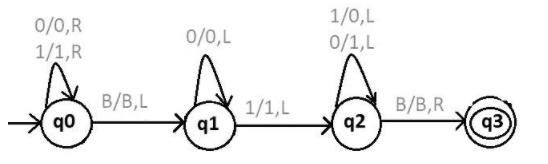# Draw a Turing machine to find 2’s complement of a binary number

2’s complement of binary numbers can be done by using two approaches.

• Traverse bits from left to right, find the 1st 1 bit then reverse all the bits after the 1 bit.

## Example

Let the input be 1110010

Thus, after performing 2’s complement, the output will be as follows −

Output − 0001110

Coming to the Turing machine to find 2’s complement,

If input is as follows −

 B 0 1 0 0 0 0 1 0 0

The output is as follows −

 B 1 0 1 1 1 1 1 0 0

## Explanation

Step 1 − Here, we need to start from the rightmost ends.

Step 2 − We will move the R/W head all the way to the right, skipping all the 0s and 1s.

Step 3 − On moving right, when we end up on the blank B, then move one step to the left.

Step 4 − Then we will move the R/W head to the left skipping all the 0s.

Step 5 − When it reaches a 1, then we will skip this 1, and then move one step left.

Step 6 − From now, we will make all the 1s to 0s and 0s to 1s.

Step 7 − We will repeat it all the way to the left of the string.

Step 8 − On moving all the way to the left, we will reach the blank B,

Step 9 − then move one step right, such that the R/W head will point to the first character, then we will stop.

The corresponding Turing machine (TM) is shown below. Here Q0 is the initial state and Q3 is the final state.## Explanation

With the help of state ‘q0’ we can reach the end of the string, whenever BLANK is reached then move towards left.

With the help of state ‘q1’ we can pass all 0’s and move left first 1 is found.

Pass single ‘1’ and then move left.

With the help of state ‘q2’ we can complement each digit and move left, whenever BLANK is reached then move towards right and reach the final state q2.